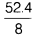# Density of material in g/cc

Given, mass of the body = 52.4 g, reading of water level in measuring cylinder without solid body (V1) = 15.4 mL, V2 (with solid body) = 23.4 mL. Then, based on above obserbation, the density of material in g/cc would be

We know that, DHere, V = V2 – V1 = 23.4 – 15.4 = 8.0 cc
∴ D =g/cc = 6.55 g/cc